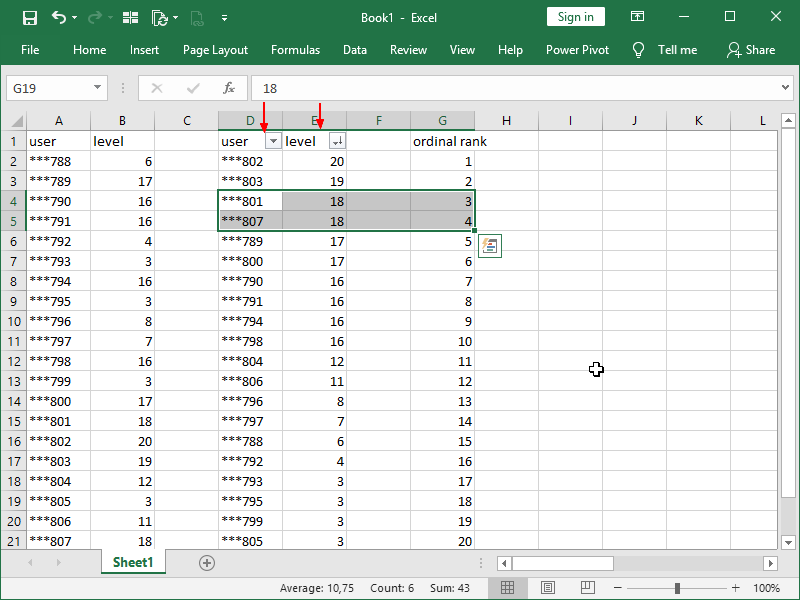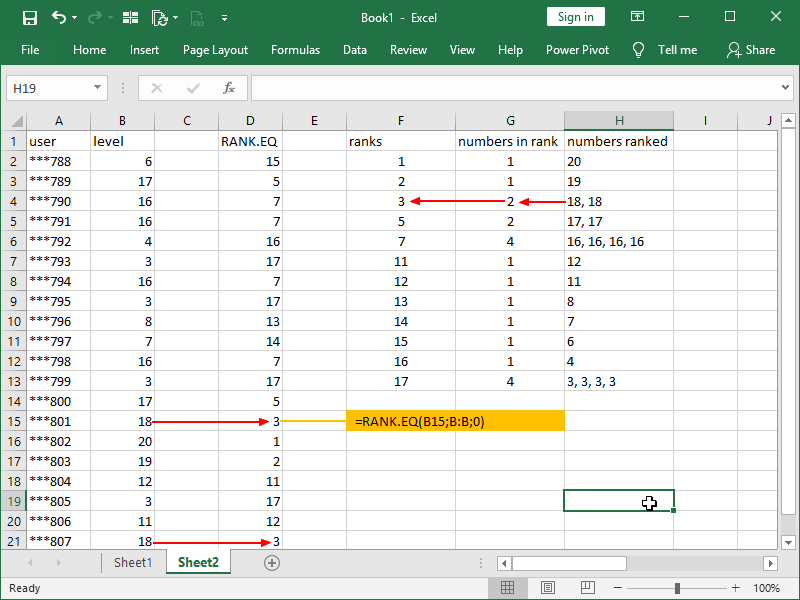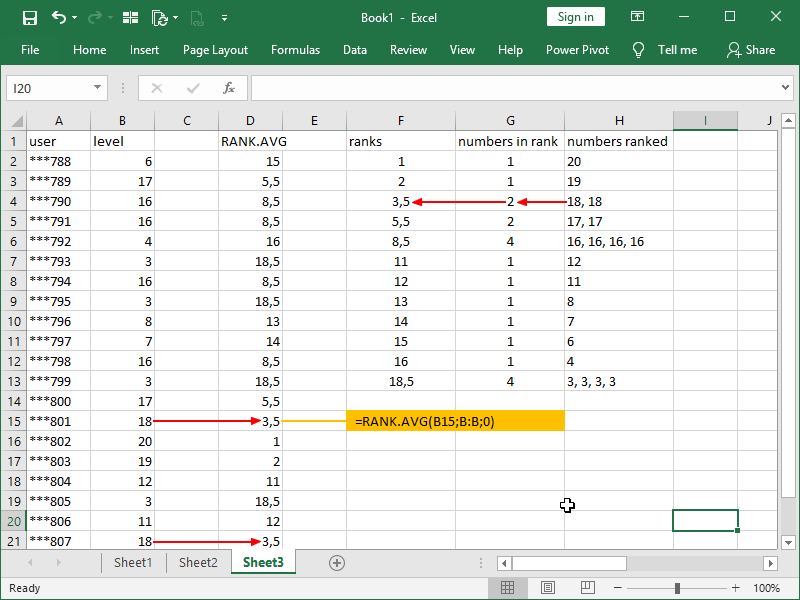# RANK functions

Ranking is data transformation in which values are replaced by their rank when the data is sorted.

For any two items, the first is either

• ranked higher than
• ranked lower than
• ranked equal to

the second.

Values can be ranked from the smallest, or from the largest.

Ordinal ranking presumes that all items will receive distinct ordinal numbers, including items that compare equal. Distinct ordinal numbers for items that are equal can be assigned arbitrarily, but it is also desirable for the ranking to be consistent (and if needed, incorporates other attributes into the ranking order) so that the results could be replicated.

Consider this example:We are ranking users, with the highest-level user ranking 1st. In order to do that we can Sort Largest to Smallest our users by level, and then assign them rank. However, in order to ensure consistency, we are first sorting our user’s codes from A to Z in the column D, then sorting our user levels largest to smallest in the column E, and only then are we assigning them rank in the column G. Our user is than always ranked higher than the other user if he is higher level than the other user, and if they are the same level, the one with the earlier user code is ranked higher.

Competition ranking presumes that items that compare equal will receive the same ranking number, and a gap is left in the ranking numbers.

The RANK.EQ function ranks numbers under competition ranking.

The RANK function is an older version of the RANK.EQ function and performs the same way.

The RANK.EQ function syntax is as follows:

= RANK.EQ ( number; ref; [order])

Number is the number whose rank you want to find.

Ref is an array of, or a reference to, a list of numbers. Non-numeric values in ref are ignored.

Order is a number specifying how to rank number.

If order is zero or omitted, Excel ranks numbers from highest to lowest, i.e. as if ref were a list sorted in descending order.

If order is 1 (or any nonzero value), Excel ranks number from lowest to highest, i.e. as if ref were a list sorted in ascending order.

Consider the following example:Both users at the level 18 are now ranked 3rd, with no one ranking 4th.

Fractional ranking also presumes that items that compare equal will receive the same ranking number. However, in this case, the ranking number will be a mean of ranking that the numbers would have under ordinal ranking.

The RANK.AVG function ranks numbers under fractional ranking.

The RANK.AVG function syntax is as follows:

= RANK.AVG ( number; ref; [order] )

Number is the number whose rank you want to find.

Ref is an array of, or a reference to, a list of numbers. Non-numeric values in ref are ignored.

Order is a number specifying how to rank number.

If order is zero or omitted, Excel ranks numbers from highest to lowest, i.e. as if ref were a list sorted in descending order.

If order is 1 (or any nonzero value), Excel ranks number from lowest to highest, i.e. as if ref were a list sorted in ascending order.

Consider the following example:Both users at the level 18 are now ranked at 3,5.

Note the difference – in our example, sum of all ordinal ranks is 210, sum of all competitive ranks is 196 and sum of all fractional ranks is 210 – the same as the sum of all ordinal ranks.

Dig deeper:

Numbers in Excel